# ISEE Middle Level Math : How to find a ratio

## Example Questions

1 3 Next →

### Example Question #21 : How To Find A Ratio

A boardgame has 22 pieces. Half of the pieces are blue and the other half are red. John has hidden 4 red pieces and 3 blue pieces. Of the remaining pieces, what is the ratio of red pieces to blue pieces?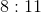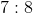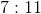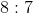Explanation:

If there is a boardgame in which there are 22 pieces, half of which are blue and half of which are red, this means that there are 11 blue pieces and 11 red pieces. If John has hidden 4 red pieces and 3 blue pieces, there are therefore 7 red pieces remaining and 8 blue pieces remaining.

Thus, the ratio of red pieces to blue pieces (of what remains) is.

### Example Question #901 : Isee Middle Level (Grades 7 8) Mathematics Achievement

The ratio of cats to dogs in the pet store is 2:3.  If there are 21 dogs, how many more dogs are there than cats?Explanation:

Set up a proportion and cross multiply to determine the number of cats.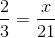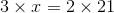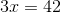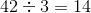Then find the difference between the number of dogs and cats by using subtraction.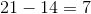### Example Question #21 : Numbers And Operations

If you want to share a pizza with your friend with a ratio of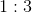with you gettingpart and your friend gettingparts, how much pizza does your friend get if there areslices of pizza?Explanation:

If the ratio is, there aretotal parts.

That means your friend getout ofparts or.

If a pizza hasslices, that means that your friend's number of slices isoforslices.

### Example Question #11 : Grade 7

A car travels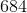miles inhours. At what rate does the car travel?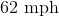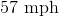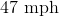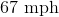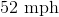Explanation:

Divide the number of miles by the number of hours to get the number of miles per hour.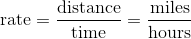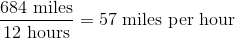### Example Question #22 : Numbers And Operations

Simplify the ratio: 8.5 to 2.5

19 to 5

16 to 5

18 to 5

17 to 5

17 to 5

Explanation:

8.5 to 2.5 can be rewritten and simplified as follows: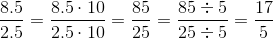The simplified form of the ratio is 17 to 5.

### Example Question #23 : Numbers And Operations

Billy has six horses and Susie has fifteen horses. What is the appropriate ratio of Susie's horses to Billy's horses?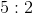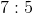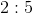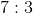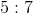Explanation:

Use the colon symbol to represent the ratio.  If we have a ratio of A to B, then this can be rewritten as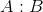.

Write the ratio of Susie's horses to Billy's horses.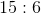We can reduce this like fractions.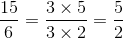This means that the ratio can also be:The answer is:1 3 Next →

### All ISEE Middle Level Math Resources